# Wavefunction Quantum mechanics acknowledges the waveparticle duality of

• Slides: 45Wavefunction • Quantum mechanics acknowledges the waveparticle duality of matter by supposing that, rather than traveling along a definite path, a particle is distributed through space like a wave. The wave that in quantum mechanics replaces the classical concept of particle trajectory is called a wavefunction, ψ (“psi”).A wave function in quantum mechanics describes the quantum state of an isolated system of one or more particles. There is one wave function containing all the information about the entire system, not a separate wave function for each particle in the system.Wave equation for the harmonic motion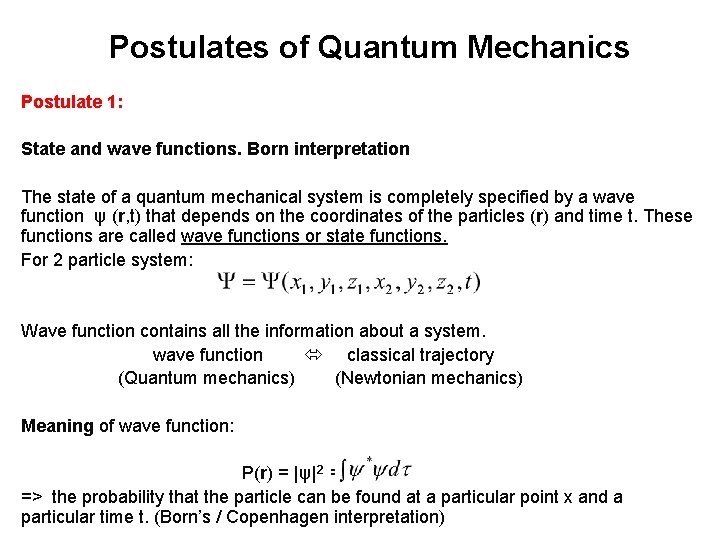Postulates of Quantum Mechanics Postulate 1: State and wave functions. Born interpretation The state of a quantum mechanical system is completely specified by a wave function ψ (r, t) that depends on the coordinates of the particles (r) and time t. These functions are called wave functions or state functions. For 2 particle system: Wave function contains all the information about a system. wave function classical trajectory (Quantum mechanics) (Newtonian mechanics) Meaning of wave function: P(r) = |ψ|2 = => the probability that the particle can be found at a particular point x and a particular time t. (Born’s / Copenhagen interpretation)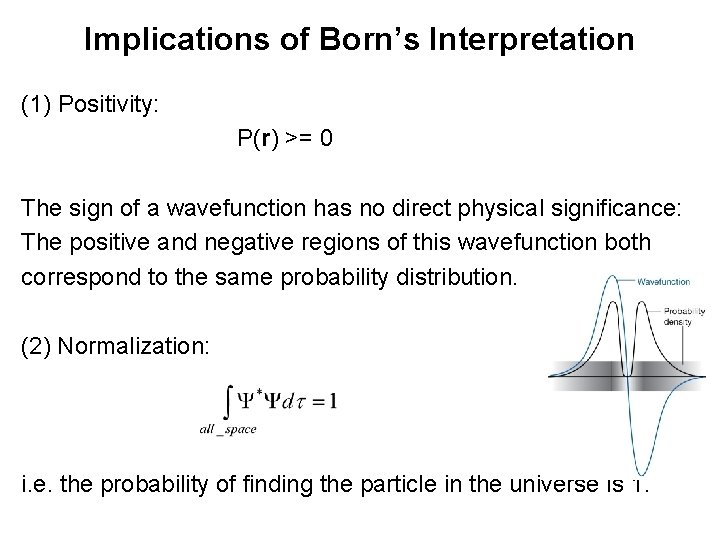Implications of Born’s Interpretation (1) Positivity: P(r) >= 0 The sign of a wavefunction has no direct physical significance: The positive and negative regions of this wavefunction both correspond to the same probability distribution. (2) Normalization: i. e. the probability of finding the particle in the universe is 1.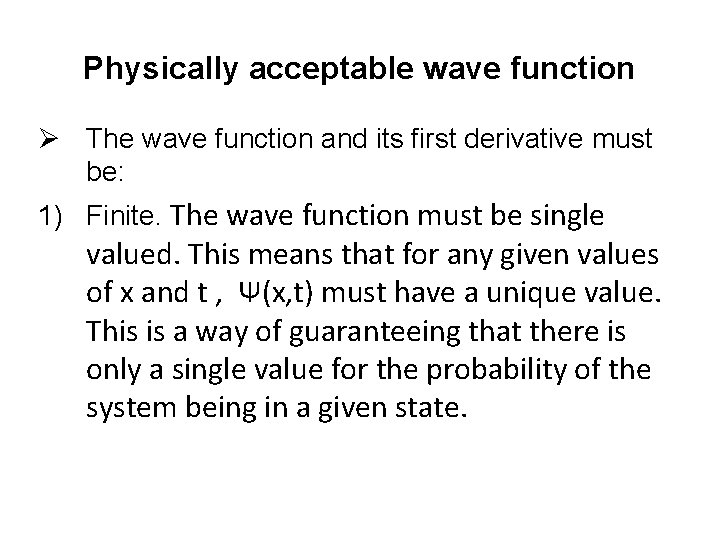Physically acceptable wave function Ø The wave function and its first derivative must be: 1) Finite. The wave function must be single valued. This means that for any given values of x and t , Ψ(x, t) must have a unique value. This is a way of guaranteeing that there is only a single value for the probability of the system being in a given state.2. Square-integrable The wave function must be square-integrable. In other words, the integral of |Ψ|2 over all space must be finite. This is another way of saying that it must be possible to use |Ψ|2 as a probability density, since any probability density must integrate over all space to give a value of 1, which is clearly not possible if the integral of |Ψ|2 is infinite. One consequence of this proposal is that must tend to 0 for infinite distances.Continuous wavefunction • A rapid change would mean that the derivative of the function was very large (either a very large positive or negative number). In the limit of a step function, this would imply an infinite derivative. Since the momentum of the system is found using the momentum operator, which is a first order derivative, this would imply an infinite momentum, which is not possible in a physically realistic system.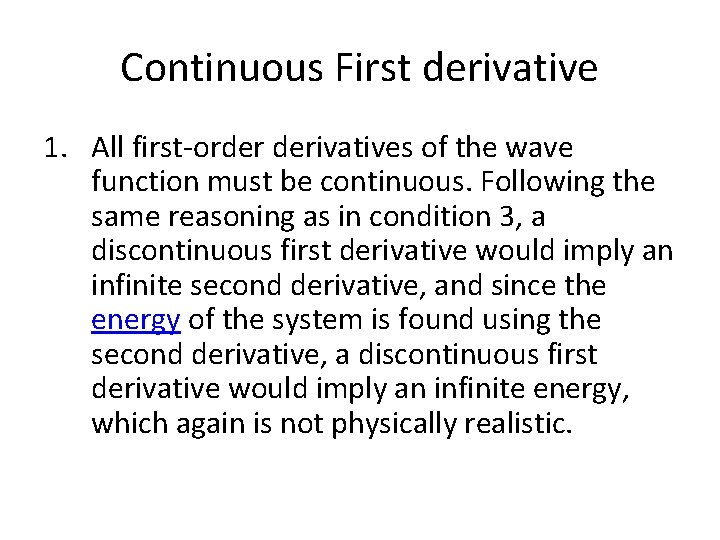Continuous First derivative 1. All first-order derivatives of the wave function must be continuous. Following the same reasoning as in condition 3, a discontinuous first derivative would imply an infinite second derivative, and since the energy of the system is found using the second derivative, a discontinuous first derivative would imply an infinite energy, which again is not physically realistic.Acceptable or Not ? ?Acceptable or not acceptable ? ?Exp(x)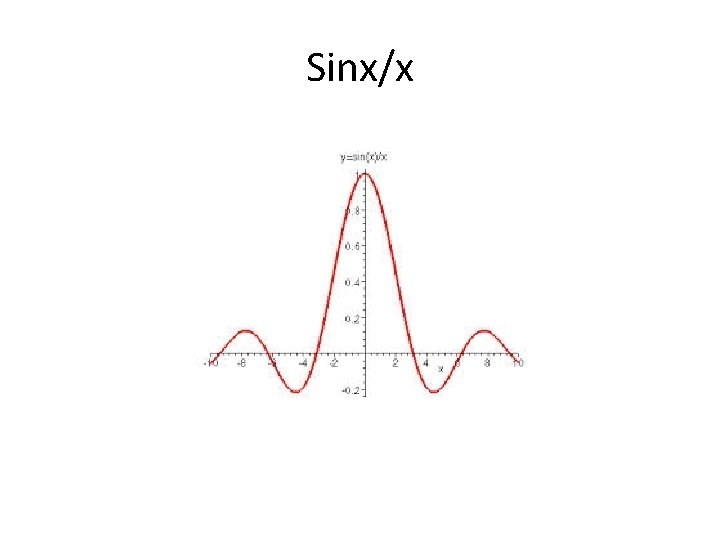Sinx/xSin-1 x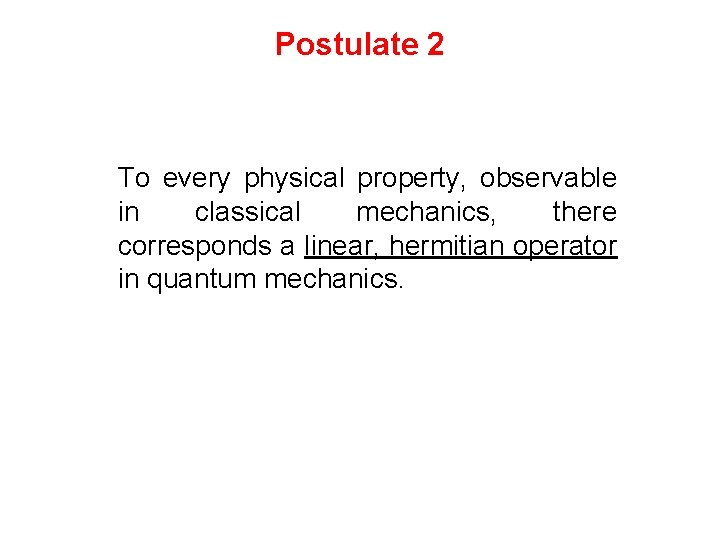Postulate 2 To every physical property, observable in classical mechanics, there corresponds a linear, hermitian operator in quantum mechanics.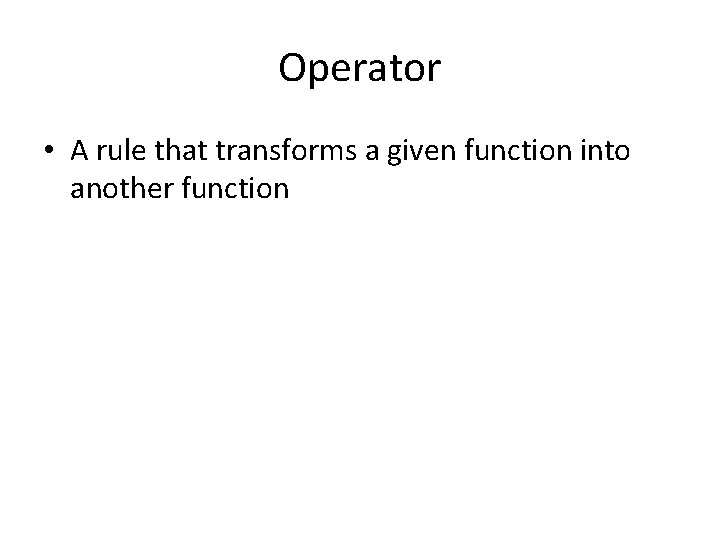Operator • A rule that transforms a given function into another functionOperator • Example. Apply the following operators on the given functions: • (a) Operator d/dx and function x 2. • (b) Operator d 2/dx 2 and function 4 x 2. • (c) Operator (∂/∂y)x and function xy 2. • (d) Operator −iћd/dx and function exp(−ikx). • (e) Operator −ћ 2 d 2/dx 2 and function exp(−ikx).Identifying the operatorsHermitian Operator Hermitian operators have two properties that forms the basis of quantum mechanics (i) Eigen value of a Hermitian operator are real. (ii) Eigenfunctions of Hermitian operators are orthogonal to each other or can be made orthogonal by taking linear combinations of them. •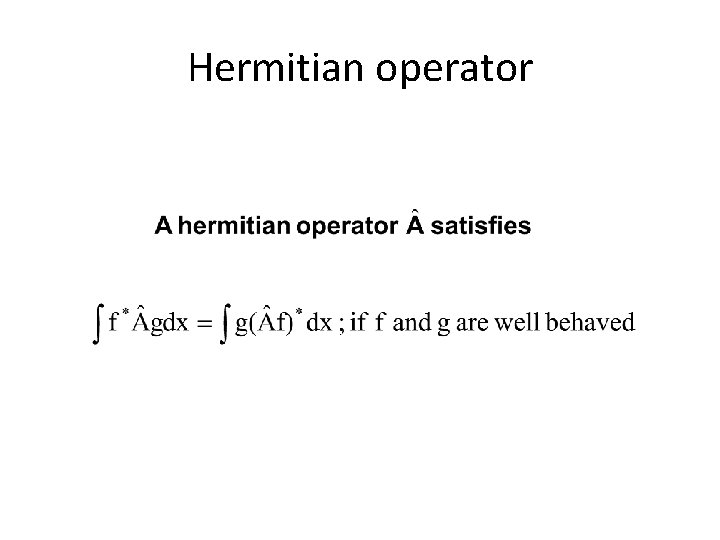Hermitian operator• Prove Operator x is Hermitian.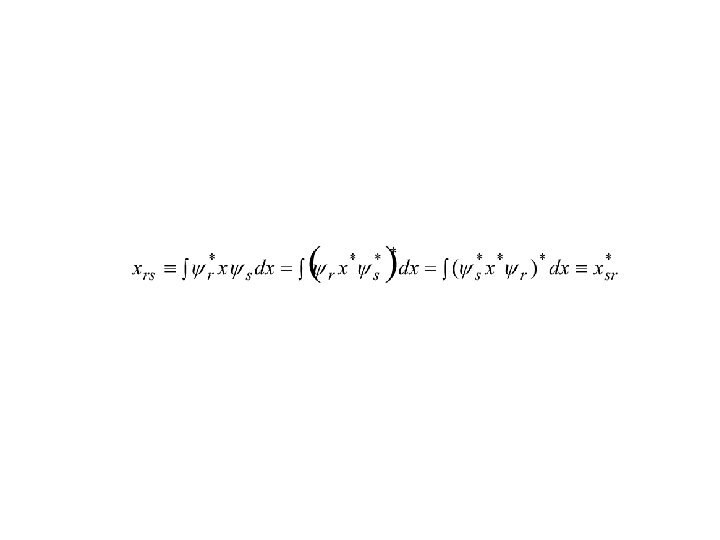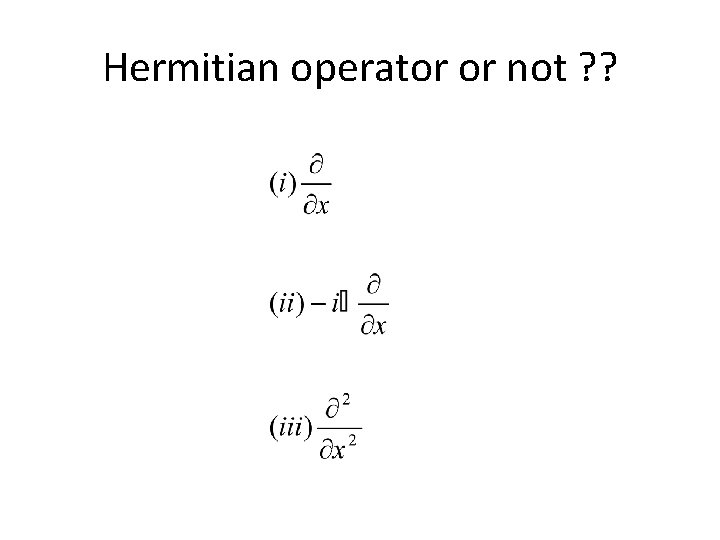Hermitian operator or not ? ?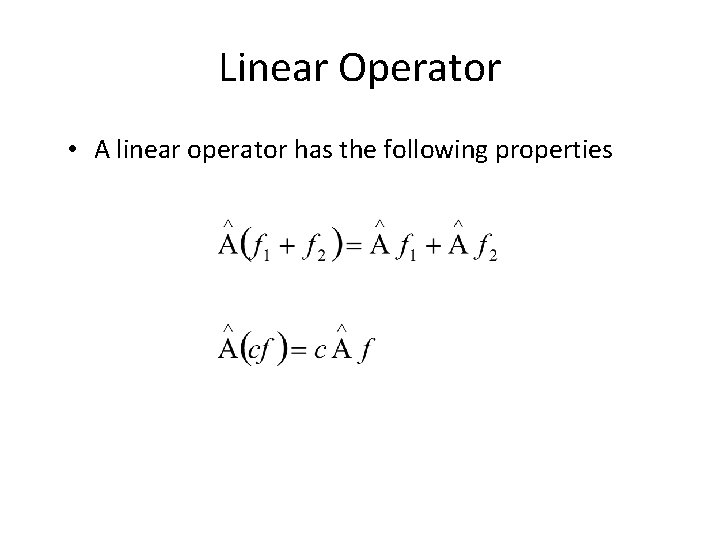Linear Operator • A linear operator has the following properties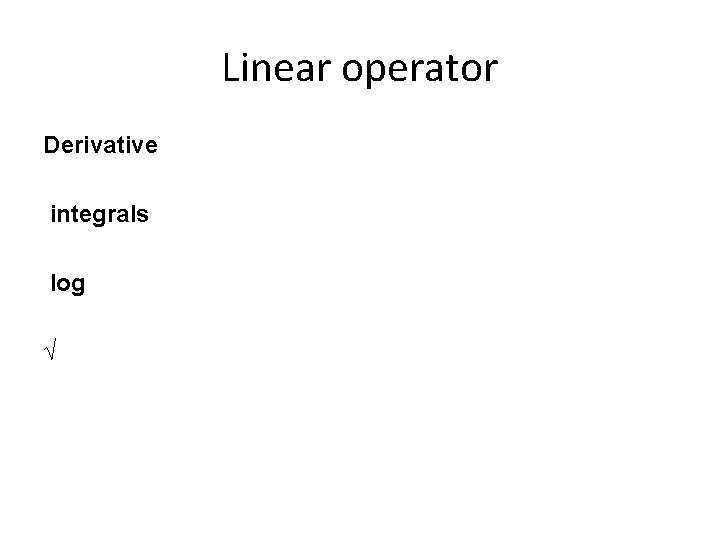Linear operator Derivative integrals log √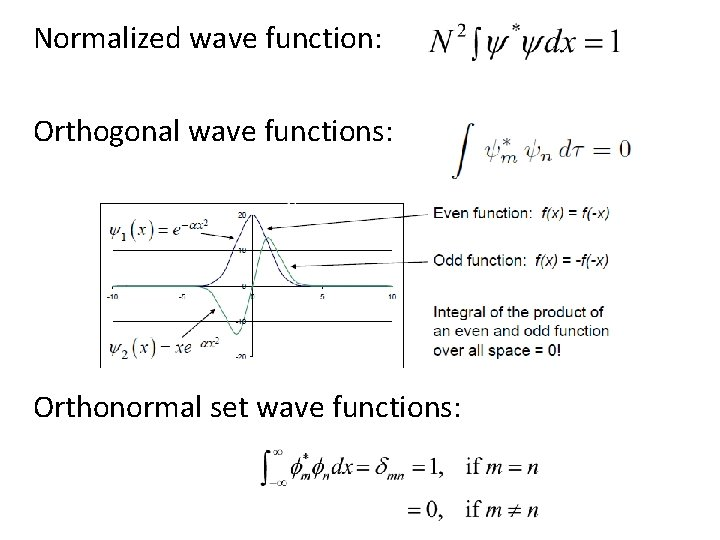Normalized wave function: Orthogonal wave functions: Orthonormal set wave functions: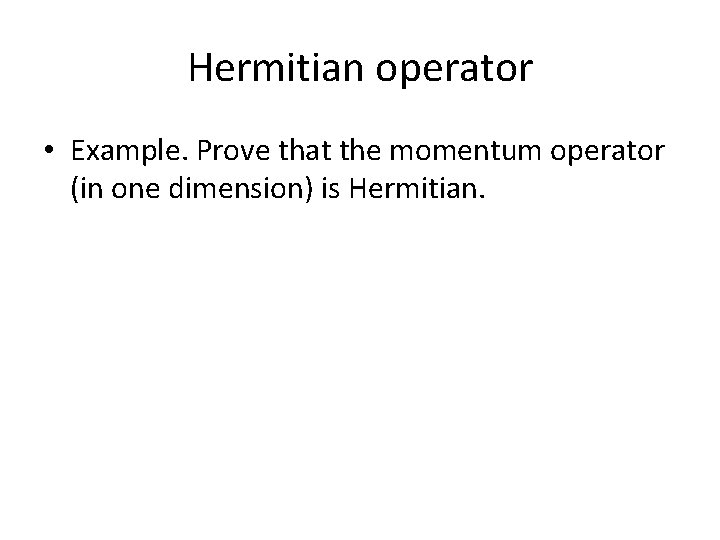Hermitian operator • Example. Prove that the momentum operator (in one dimension) is Hermitian.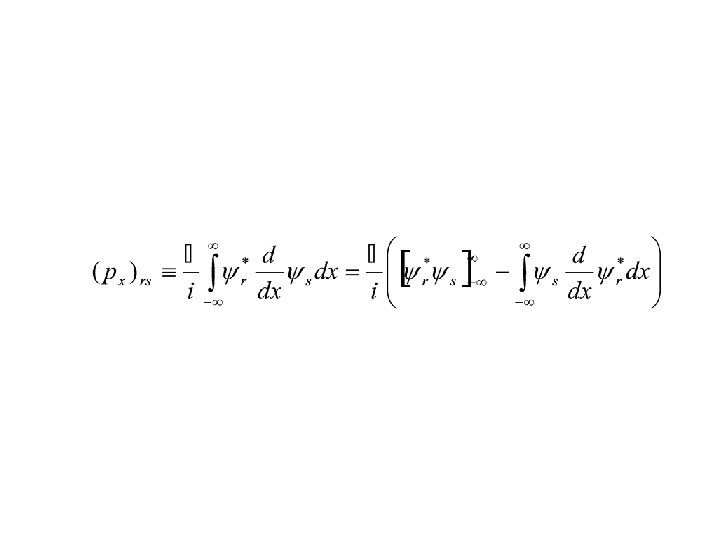Postulate 3 In any measurement of the observable associated with the operator , the only values that will ever be observed are the eigenvalues ‘a’ which satisfy the eigenvalue equation: This is the postulate that the values of dynamical variables are quantized in quantum mechanics.Eigen Function and Eigen value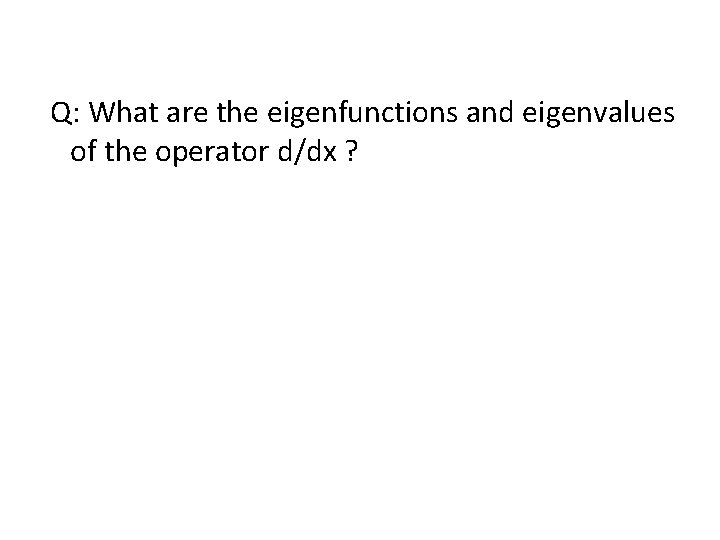Q: What are the eigenfunctions and eigenvalues of the operator d/dx ?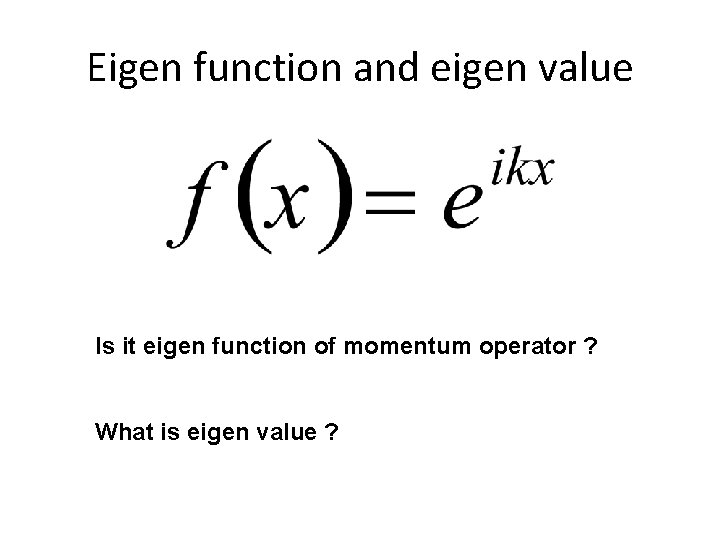Eigen function and eigen value Is it eigen function of momentum operator ? What is eigen value ?Eigenvalue equation (Operator)(function) = (constant factor)*(same function) Example: eikx is an eigenfunction of a operator F(x) = eikx Thus eikx is an eigenfunctionSignificance of commutation rules • The eigenvalue of a Hermitian operator is real. • A real eigenvalue means that the physical quantity for which the operator stands for can be measured experimentally. • The eigenvalues of two commuting operators can be computed by using the common set of eigenfunctions. If the two operators commute, then it is possible to measure the simultaneously the precise value of both the physical quantities for which the operators stand for. Question: Find commutator of the operators x and px Is it expected to be a non-zero or zero quantity? Hint: Heisenberg Uncertainty PrincipleCommute or not ? ? • Operator x and d/dx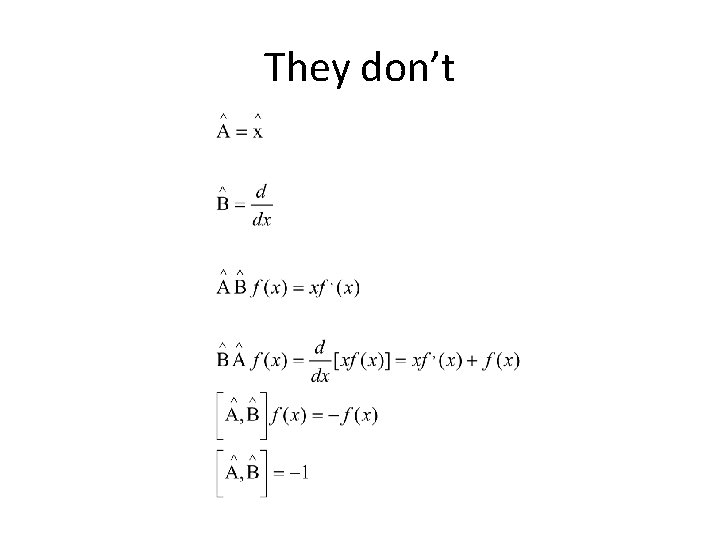They don’t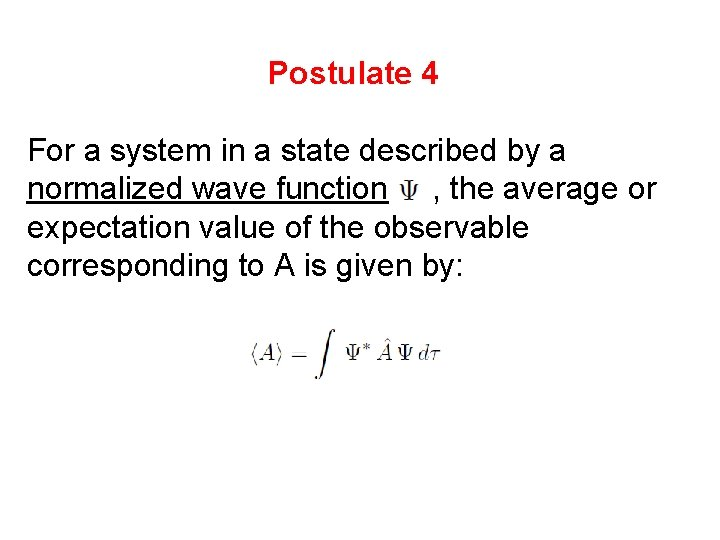Postulate 4 For a system in a state described by a normalized wave function , the average or expectation value of the observable corresponding to A is given by: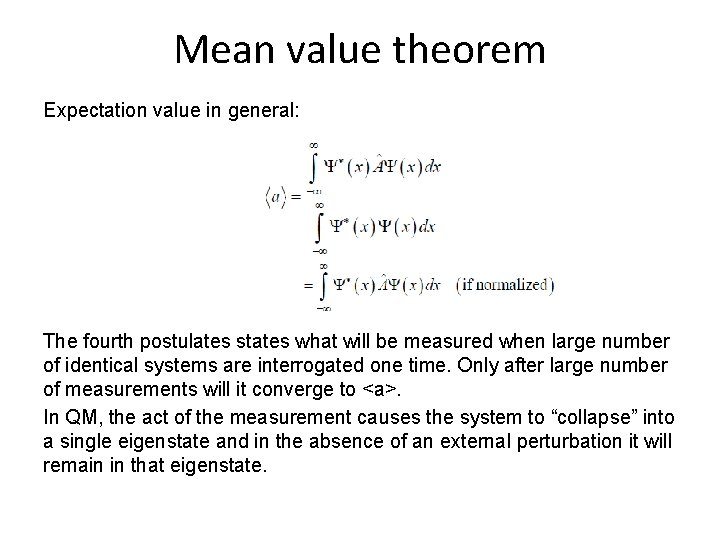Mean value theorem Expectation value in general: The fourth postulates states what will be measured when large number of identical systems are interrogated one time. Only after large number of measurements will it converge to <a>. In QM, the act of the measurement causes the system to “collapse” into a single eigenstate and in the absence of an external perturbation it will remain in that eigenstate.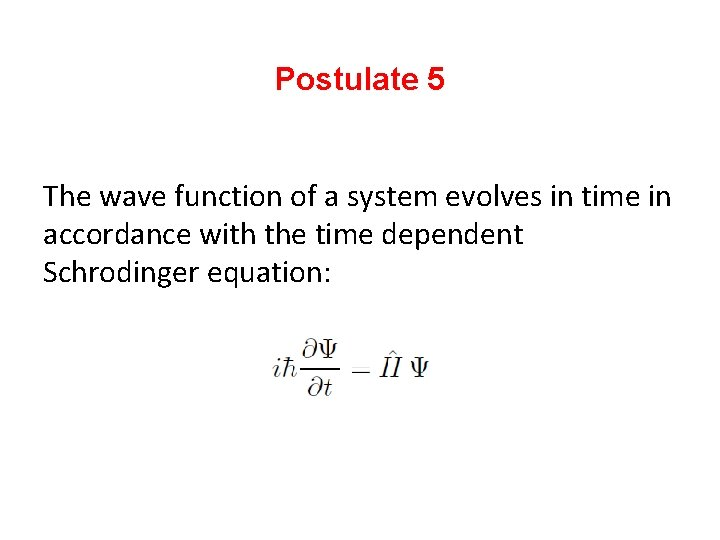Postulate 5 The wave function of a system evolves in time in accordance with the time dependent Schrodinger equation: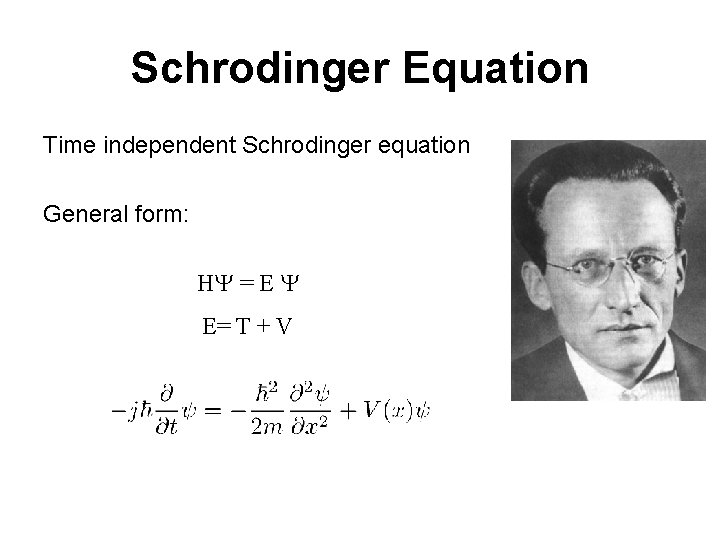Schrodinger Equation Time independent Schrodinger equation General form: H = E E= T + VSchrödinger Representation – Schrödinger Equation Time dependent Schrödinger Equation Developed through analogy to Maxwell’s equations and knowledge of the Bohr model of the H atom. Sum of kinetic energy and potential energy. Hamiltonian Q. M. kinetic potential energy one dimension recall three dimensions The potential, V, makes one problem different form another H atom, harmonic oscillator. Copyright – Michael D. Fayer, 2007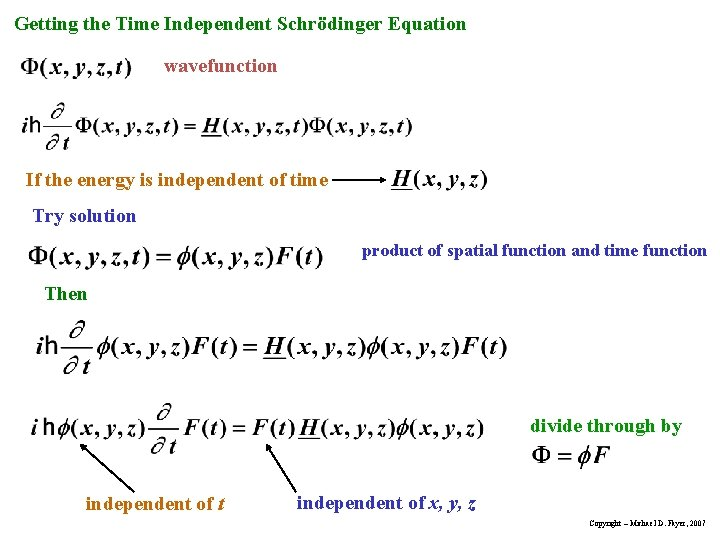Getting the Time Independent Schrödinger Equation wavefunction If the energy is independent of time Try solution product of spatial function and time function Then divide through by independent of t independent of x, y, z Copyright – Michael D. Fayer, 2007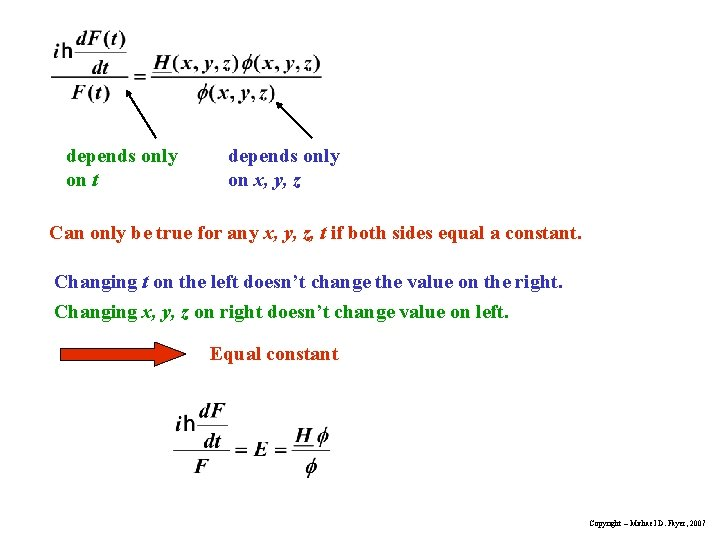depends only on t depends only on x, y, z Can only be true for any x, y, z, t if both sides equal a constant. Changing t on the left doesn’t change the value on the right. Changing x, y, z on right doesn’t change value on left. Equal constant Copyright – Michael D. Fayer, 2007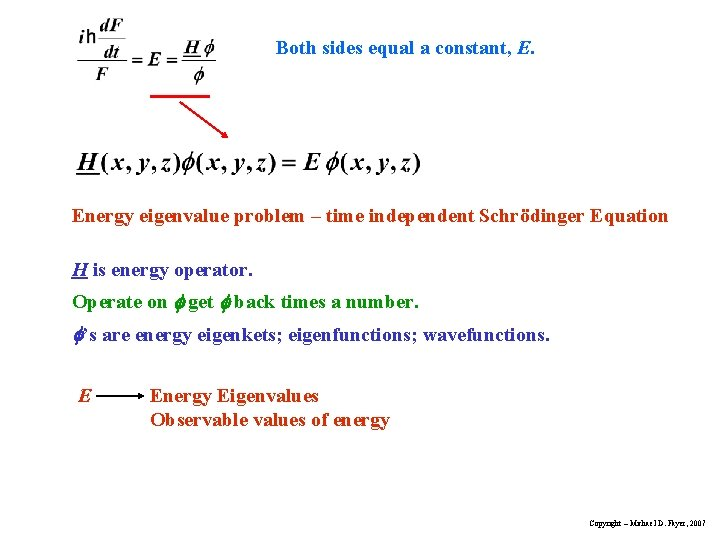Both sides equal a constant, E. Energy eigenvalue problem – time independent Schrödinger Equation H is energy operator. Operate on f get f back times a number. f’s are energy eigenkets; eigenfunctions; wavefunctions. E Energy Eigenvalues Observable values of energy Copyright – Michael D. Fayer, 2007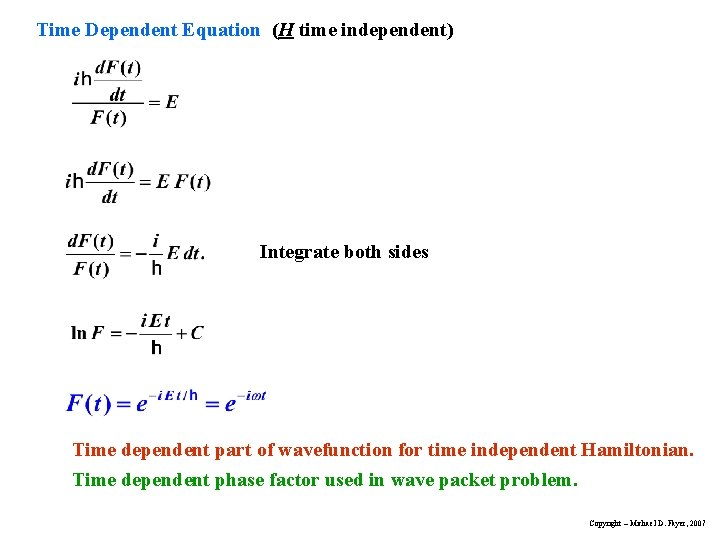Time Dependent Equation (H time independent) Integrate both sides Time dependent part of wavefunction for time independent Hamiltonian. Time dependent phase factor used in wave packet problem. Copyright – Michael D. Fayer, 2007